## 3. The Perrin sequence

The analogy between the golden number Φ and the plastic number P led mathematicians to investigate whether even for P it was possible to define a sequence analogous to that of Fibonacci, ie a sequence of natural numbers from which to extract a sequence of fractions converging to P.

A first solution to the problem, proposed by R. Perrin and therefore known as the Perrin sequence, can be found in the following way.

Let α, β and γ, where α is real and β e γ complex conjugates with norm < 1, be the roots of the equation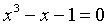that is numbers such that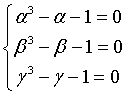and let pi be the sum of their powers with exponent i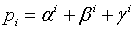you immediately have p0 = 3.

Moreover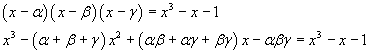Since the polynomials must be identical, you have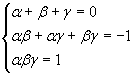The sum of the first powers of the roots is equal to 0, so p1 = 0.

The sum of the squares of the roots can be obtained in the following way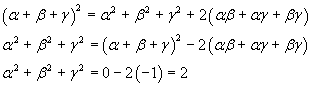The sum of the squares of the roots is equal to 2, so p2 = 2.

The sum of the cubes of the roots can be obtained in the following way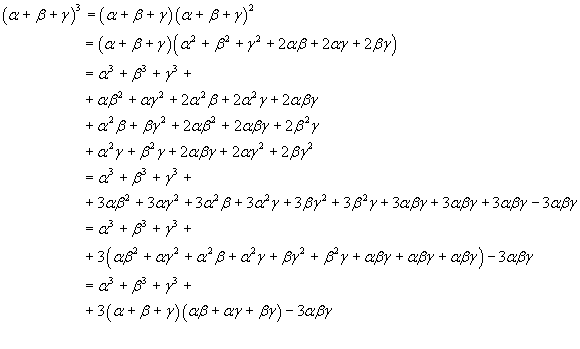You have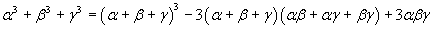and, since the sum of the roots is 0 and their product is 1,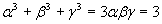The sum of the cibes of the roots is 3, so p3 = 3.

Moreover you can observe that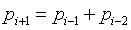that is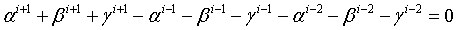because the sum of the powers of α is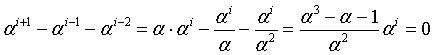and the same holds for the sums of the powers of β and γ.

Therefore, given p0, p1, p2 and p3, you can obtain all the terms pi of the sequence

 i 0 1 2 3 4 5 6 7 8 9 10 11 12 13 14 15 16 17 18 19 20 ... pi 3 0 2 3 2 5 5 7 10 12 17 22 29 39 51 68 90 119 158 209 277 ...

This is the Perrin sequence; its terms are the Perrin numbers.

The use of the recursive definition for the computer calculation of the n-th Perrin is impractical. Better to use a loop, as in the following Javascript implementation

```function nPerrin(n)
{
n = parseInt(n);
var n1, n2, n3, p;
switch(n)
{
case 0: return 3;
case 1: return 0;
case 2: return 2;
default:
n1 = 2;
n2 = 0;
n3 = 3;
for (var i=3; i <= n; i++)
{
p = ( n2 + n3 );
n3 = n2;
n2 = n1;
n1 = p;
}
return(p);
}
}
```

### Perrin numbers

index i

number

If you can use Mathematica (Wolfram), you may define the following function

```PerrinN[n_]:=Switch[n,0,3,1,0,2,2,_,Module[{i,n1,n2,n3,val},n1=2;n2=0;n3=3;
For[i=3,i <= n,i++,val=n2+n3;n3=n2;n2=n1;n1=val];val]]
Table[PerrinN[n],{n,0,30}]
{3,0,2,3,2,5,5,7,10,12,17,22,29,39,51,68,90,119,158,209,277,367,486,644,853,1130,1497,1983,2627,3480,4610}
```

The Perrin sequence, as it was proposed, has the following property.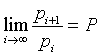Infact, if α is the real root, that is P, and β and γ are conjugate complex numbers with norm < 1, their powers converge to 0 as the exponent increases. So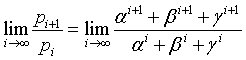By dividing numerator and denominator by αi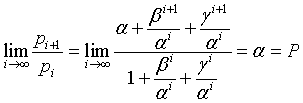The French mathematician E. Lucas noticed an interesting property of the Perrin numbers analyzed by him: if the index i of a termn pi of the sequence is prime, the term is exact multiple of the index. For example

 pi 3 0 2 3 2 5 5 7 10 12 17 22 29 39 51 68 90 119 158 209 277 ... i 0 1 2 3 4 5 6 7 8 9 10 11 12 13 14 15 16 17 18 19 20 ... resto 0 0 0 2 0 5 0 2 3 7 0 5 0 9 8 10 0 14 0 17 ...

Theorem: If i is prime, i is a divisor of pi.

If i is a prime number, you have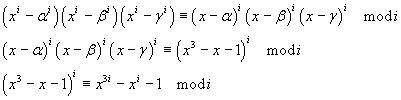Therefore, for the transitivity of the congruence modulo i you have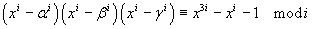Moreover you have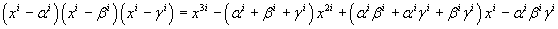The coefficients of x2i must b equal, so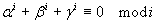that is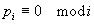Thus, if i is prime, the i-th number of Perrin is divisible by i.

But this does not imply the inverse theorem: you cannot say that if the n-th Perrin number is divisible by n then n is prime.

If the inverse theorem were valid, it would offer a valid primality test: to know if a number is prime you check whether it is an exact divisor of the corresponding Perrin number. But this conjecture has been falsified: there are numbers divisible by the corresponding index which are not prime: these numbers are called Perrin pseudoprimes.
For example, p271441 is divisible by 271441, but 271441 is not prime: it is the square of 521; 271441 is therefore a Perrin pseudoprime.

(The calculation of p271441 can be performed by the proposed JS application but may take a few minutes; if possible, try Mathematica.)